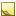﻿ SimpleShapeChecker Class# SimpleShapeChecker Class

A class for checking simple geometrical shapes.Inheritance Hierarchy
SystemObject
Accord.Math.GeometrySimpleShapeChecker

Namespace:  Accord.Math.Geometry
Assembly:  Accord.Math (in Accord.Math.dll) Version: 3.8.0Syntax
`public class SimpleShapeChecker`

The SimpleShapeChecker type exposes the following members.Constructors
NameDescriptionSimpleShapeChecker
Initializes a new instance of the SimpleShapeChecker class
TopProperties
NameDescriptionAngleError
Maximum allowed angle error in degrees, [0, 20].LengthError
Maximum allowed difference in sides' length (relative to shapes' size), [0, 1].MinAcceptableDistortion
Minimum value of allowed shapes' distortion.RelativeDistortionLimit
Maximum value of allowed shapes' distortion, [0, 1].
TopMethods
NameDescriptionCheckIfPointsFitShape
Check if a shape specified by the set of points fits a convex polygon specified by the set of corners.CheckPolygonSubType
Check sub type of a convex polygon.CheckShapeType
Check type of the shape formed by specified points.Equals
Determines whether the specified object is equal to the current object.
(Inherited from Object.)Finalize
Allows an object to try to free resources and perform other cleanup operations before it is reclaimed by garbage collection.
(Inherited from Object.)GetHashCode
Serves as the default hash function.
(Inherited from Object.)GetType
Gets the Type of the current instance.
(Inherited from Object.)IsCircle(ListIntPoint)
Check if the specified set of points form a circle shape.IsCircle(ListIntPoint, Point, Single)
Check if the specified set of points form a circle shape.IsConvexPolygon
Check if the specified set of points form a convex polygon shape.IsQuadrilateral(ListIntPoint)
Check if the specified set of points form a quadrilateral shape.IsQuadrilateral(ListIntPoint, ListIntPoint)
Check if the specified set of points form a quadrilateral shape.IsTriangle(ListIntPoint)
Check if the specified set of points form a triangle shape.IsTriangle(ListIntPoint, ListIntPoint)
Check if the specified set of points form a triangle shape.MemberwiseClone
Creates a shallow copy of the current Object.
(Inherited from Object.)ToString
Returns a string that represents the current object.
(Inherited from Object.)
TopExtension Methods
NameDescriptionHasMethod
Checks whether an object implements a method with the given name.
(Defined by ExtensionMethods.)IsEqual
Compares two objects for equality, performing an elementwise comparison if the elements are vectors or matrices.
(Defined by Matrix.)To(Type)Overloaded.
Converts an object into another type, irrespective of whether the conversion can be done at compile time or not. This can be used to convert generic types to numeric types during runtime.
(Defined by ExtensionMethods.)ToTOverloaded.
Converts an object into another type, irrespective of whether the conversion can be done at compile time or not. This can be used to convert generic types to numeric types during runtime.
(Defined by ExtensionMethods.)
TopRemarks

The class performs checking/detection of some simple geometrical shapes for provided set of points (shape's edge points). During the check the class goes through the list of all provided points and checks how accurately they fit into assumed shape.

All the shape checks allow some deviation of points from the shape with assumed parameters. In other words it is allowed that specified set of points may form a little bit distorted shape, which may be still recognized. The allowed amount of distortion is controlled by two properties (MinAcceptableDistortion and RelativeDistortionLimit), which allow higher distortion level for bigger shapes and smaller amount of distortion for smaller shapes. Checking specified set of points, the class calculates mean distance between specified set of points and edge of the assumed shape. If the mean distance is equal to or less than maximum allowed distance, then a shape is recognized. The maximum allowed distance is calculated as:

`maxDistance = max( minAcceptableDistortion, relativeDistortionLimit * ( width + height ) / 2 )`
, where width and height is the size of bounding rectangle for the specified points.

See also AngleError and LengthError properties, which set acceptable errors for polygon sub type checking done by CheckPolygonSubType(ListIntPoint) method.Note
See the next article for details about the implemented algorithms: Detecting some simple shapes in images.

Sample usage:

```private List<IntPoint> idealCicle = new List<IntPoint>( );
private List<IntPoint> distorredCircle = new List<IntPoint>( );
System.Random rand = new System.Random( );

// generate sample circles
float radius = 100;

for ( int i = 0; i < 360; i += 10 )
{
float angle = (float) ( (float) i / 180 * System.Math.PI );

// add point to ideal circle
(int) ( radius * System.Math.Cos( angle ) ),
(int) ( radius * System.Math.Sin( angle ) ) ) );

// add a bit distortion for distorred cirlce
float distorredRadius = radius + rand.Next( 7 ) - 3;

(int) ( distorredRadius * System.Math.Cos( angle ) ),
(int) ( distorredRadius * System.Math.Sin( angle ) ) ) );
}

// check shape
SimpleShapeChecker shapeChecker = new SimpleShapeChecker( );

if ( shapeChecker.IsCircle( idealCicle ) )
{
// ...
}

if ( shapeChecker.CheckShapeType( distorredCircle ) == ShapeType.Circle )
{
// ...
}```See Also